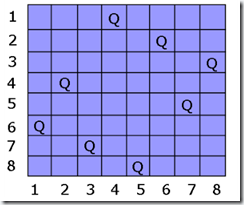基础算法系列总结：回溯算法（解火力网问题）

1、定义一个解空间，它包含问题的解。

2、利用适于搜索的方法组织解空间。

3、利用深度优先法搜索解空间。

4、利用限界函数避免移动到不可能产生解的子空间。1、从空棋盘起，逐行放置棋子。
2、每在一个布局中放下一个棋子，即推演到一个新的布局。
3、如果当前行上没有可合法放置棋子的位置，则回溯到上一行，重新布放上一行的棋子。View Code
#include <stdio.h>
#include
<math.h>

#include
<stdlib.h>

static int n,x[1000
];
static    long
sum;
int Place(int
k)

for(int j=1;j <k; j++

if((abs(k-j) == abs(x[j]-x[k]))||(x[j]==x[k])) return 0

return 1

}

void Backtrak(int
t)

if(t>n) sum++

else

for(int i=1; i <= n; i++

{
x[t]
=
i;

if(Place(t))Backtrak(t+1
);
}
}

int
main()

int
nn;

while(scanf("%d",&nn)!=
EOF)
{
n
=
nn;
sum
=0

for(int i=0;i<=n;i++

x[i]
=0

Backtrak(
1
);
printf(
"%d\n"
,sum);

}输入输出示例
Sample input:

4
——————输入的n值
.X..

....

XX..

....

2

XX
.X
3

.X.
X.X
.X.
4

....
....
....
....

Sample output:

5

1

5

4View Code
#include <stdio.h>

char map;

int best,n;

int canput(int row, int col)
{

int i;

for (i = row - 1; i >= 0; i--
{

if (map[i][col] == 'o'return 0

if (map[i][col] == 'x'break;
}

for (i = col - 1; i >= 0; i--)
{

if (map[row][i] == 'o'return 0

if (map[row][i] == 'x'break;
}

return 1;
}

void solve(int k,int tot)
{

int x,y;

if(k==n*n)
{

if(tot>best)
{
best
=tot;   return;
}
}

else
{
x
=k/n;
y
=k%n;

if((map[x][y]=='.'&& (canput(x,y) ) )
{
map[x][y]
='o';
solve(k
+1,tot+1);
map[x][y]
='.';
}
solve(k
+1,tot);
}
}

int main()
{

int i,j;
scanf(
"%d",&n);

while(n>0)
{

for(i=0;i< n;i++)

for(j=0;j< n;j++)
scanf(
"%1s",&map[i][j]);
best
=0;
solve(
0,0);
printf(
"%d\n",best);
n
=0;
scanf(
"%d",&n);
}

return 0;
}

对上面的代码做一下点解释，canput是做检验的，检验放在某个地点到底行不行得通，solve才是真正进行递归回溯的函数。。

算法系列目录：

posted @ 2011-05-20 21:33  大熊先生|互联网后端技术  阅读(12518)  评论(4编辑  收藏  举报